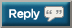# Thread: Let's OHP 2,020,000 lbs in 2020

1. + 2750 = 1,720,339

Hallelujah the gyms are now open in the England!!
High volume day for me:

1,720,589 + 4,450 = 1,725,0392. High volume day for me:

1,720,589 + 4,450 = 1,725,039
7 x 35kg
7 x 35kg
7 x 35kg
7 x 35kg
7 x 35kg + 5 x 35kg (AMRAP then push press)

40 x 35kg = 1400kg (3087lb)

3087 + 1,725,039 = 1,728,1263. 7 x 35kg
7 x 35kg
7 x 35kg
7 x 35kg
7 x 35kg + 5 x 35kg (AMRAP then push press)

40 x 35kg = 1400kg (3087lb)

3087 + 1,725,039 = 1,728,126
3740 + 1,728,126 = 17318664. + 2640 = 1,734,5065. AT LAST!!! I'm back. After a long COVID layoff
2nd workout of WrongLifts 5*10. (That's Stronglifts starting from empty bar, but running 5 sets 10 as long as I can, plus pull-ups and extra upper back work)

5*10 @ 20Kg - that's right empty bar, it can only get better from here.

1,734,506+2,200 =1,736,7066. Saturday and Tuesday

2,240 + 2,320 + 1,736,706 = 1,741,2667. Saturday and Tuesday

2,240 + 2,320 + 1,736,706 = 1,741,266
+1864 = 1,743,1308.9. 3795 + 1,746,145 = 174994010. + 2640 = 1,752,58011. Another high volume day:

+ 5,500 = 1,758,08012. Another high volume day:

+ 5,500 = 1,758,080
+ 2,320 = 1,760,40013. Using DUP this phase of my program, starting with a deload:

MONDAY
5 x 35kg
8 x 35kg (AMRAP)

SATURDAY
9 x 32.5kg (2 RIR)
9 x 32.5kg (AMRAP)

No push press this time.

13 x 35kg = 455kg
18 x 32.5kg = 585kg

455kg + 585kg = 1040kg (2293lb)

2293 + 1,760,400 = 1,762,69314. 3828 + 1,762,693 = 1,766,52115. 4th session of WrongLifts, so an OHP day again. No longer empty bar, I've added a 1.25kg fract plate - now I'm lifting weights. Feel the burn!!!
5*10@22.5kg = 2,475lbs

+2475 =1,768,99616. Idiotically decided to superset my OHP with lateral raises to save time this morning. My shoulders were so fatigued from the raises that my OHP strength suffered massively.

5 x 37.5kg
5 x 37.5kg with shaky form
1 x 37.5kg (AMRAP)

11 x 37.5kg = 412.5kg (909lb)

909 + 1,768,996 = 1,769,90517. Idiotically decided to superset my OHP with lateral raises to save time this morning. My shoulders were so fatigued from the raises that my OHP strength suffered massively.

5 x 37.5kg
5 x 37.5kg with shaky form
1 x 37.5kg (AMRAP)

11 x 37.5kg = 412.5kg (909lb)

909 + 1,768,996 = 1,769,905
+ 5544 = 1,775,44918. +3,160 = 1,778,33919. WrongLifts, 1*5@20 + 5*10@25 =1,350kg
+2,970 =1,781,30920. WrongLifts, 1*5@20 + 5*10@25 =1,350kg
+2,970 =1,781,309
Another high volume day. I started GVT a couple weeks ago and I am finally getting the weight dialed in. 10x10 @ 65 supersetted with pulldowns and a 60 second rest.

+ 6500 = 1,787,80921. Another high volume day. I started GVT a couple weeks ago and I am finally getting the weight dialed in. 10x10 @ 65 supersetted with pulldowns and a 60 second rest.

+ 6500 = 1,787,809

8 x 35kg
8 x 35kg
6 x 35kg (AMRAP)

22 x 35kg = 770kg (1697lb)

1697 + 1,787,809 = 1,789,50622. WrongLifts 5*10
1*5@20 + 5*10@27.5 = 1475kg

+3,245 =1,792,75123. WrongLifts 5*10
1*5@20 + 5*10@27.5 = 1475kg

+3,245 =1,792,751
+2640 = 1,795,39124. +3,130 = 1,798,52125. 5 x 37.5kg
5 x 37.5kg
5 x 37.5kg
4 x 37.5kg (AMRAP)

19 x 37.5kg = 712.5kg (1571lb)

1571 + 1,798,521 = 1,800,092+16,960 = 920,914
You guys are already double the total on this day last year. Get 'er done!27. 5 x 37.5kg
5 x 37.5kg
5 x 37.5kg
4 x 37.5kg (AMRAP)

19 x 37.5kg = 712.5kg (1571lb)

1571 + 1,798,521 = 1,800,092
1,800,095 + 2,486 = 1,802,581lbs28. +2640 = 1,805,22129. Then you are a MAN among Men. Please add to the running total here to help the cause. All contributions appreciated30. my lifts Today

Total today 4505 + total since joining the thread 20,812 lbs
If you wish your lifts to be included in the total, please show your weights added to a running total. Everybody does their own math.

1,805,221 + 7,650 = 1,812,871#### Posting Permissions

• You may not post new threads
• You may not post replies
• You may not post attachments
• You may not edit your posts